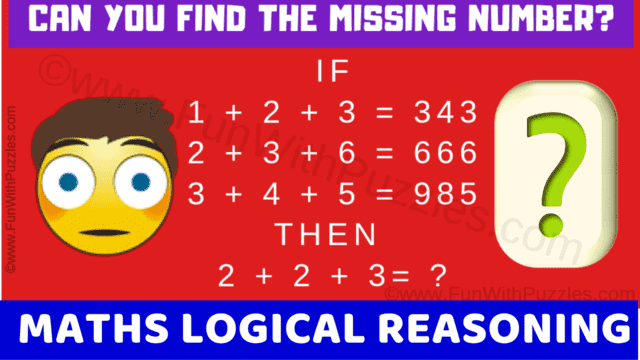This Puzzle Video contains lateral thinking questions that will make you think outside the box. In this puzzle video, there are 5 maths logic puzzles. There are some number equations in each of the puzzle questions. There is one hidden code in these equations which relates the given numbers in the equations. Your challenge is to break this hidden code and then find the value of the missing number which will solve the last equation correctly. You will need to think outside the box to solve these logical reasoning puzzle questions.
You will be given 20 seconds to solve each of these lateral thinking puzzles. If you require more time to solve any particular puzzle question, you can pause the video. Answers along with solutions to these maths logic reasoning puzzles are provided after each puzzle. Let's see how many of these puzzles you can solve correctly without looking at the answers. Write down your answer to the last bonus puzzle in the comments.